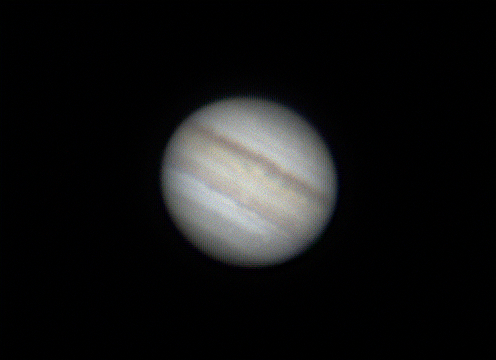Jupiter on 6/8/19Firecapture
AS3
Registax6
Gimp2

Capture Stats:

FireCapture v2.6 Settings
------------------------------------
Camera=ZWO ASI120MC-S
Filter=L
Profile=Saturn
Diameter=18.04"
Magnitude=0.22
CMI=35.9° CMIII=317.9° (during mid of capture)
FocalLength=3400mm
Resolution=0.23"
Filename=Sat_230115.ser
Date=080619
Start=230014.658
Mid=230115.058
End=230215.458
Start(UT)=060014.658
Mid(UT)=060115.058
End(UT)=060215.458
Duration=120.800s
Date_format=ddMMyy
Time_format=HHmmss
LT=UT -8h
Frames captured=4000
File type=SER
Binning=no
ROI=496x366
ROI(Offset)=368x272
FPS (avg.)=33
Shutter=30.20ms
Gain=50 (50%)
SoftwareGain=10 (off)
FPS=100 (off)
WBlue=95 (off)
USBTraffic=100 (off)
AutoExposure=off
Gamma=49 (off)
Brightness=0 (off)
WRed=52 (off)
Overclock=0 (off)
AutoGain=off
HighSpeed=off
AutoHisto=75 (off)
Histogramm(min)=0
Histogramm(max)=114
Histogramm=44%
Noise(avg.deviation)=n/a
Limit=4000 Frames
Sensor temperature=12.5°CCloudy Nights LLC Cloudy Nights Sponsor: Astronomics